PSABM5WU - Practice---Acc7.5 Lesson 11 More Solutions to Linear Equations (8.EE.C, 8.EE.C.8.a, 8.EE.C.8)
Part A)

For the following equation, find y when -3.

68

When x = -3, y = _____

Type your answer below as a number (example: 5, 3.1, 4 1/2, or 3/2):
Part B)

Now find x when 2.

When y = 2, x = _____

Type your answer below as a number (example: 5, 3.1, 4 1/2, or 3/2):
Part A)

For the following equation, find y when -3.

y =x

When x = -3, y = _____

Type your answer below as a number (example: 5, 3.1, 4 1/2, or 3/2):
Part B)

Now find x when y=2.

When y = 2, x = _____

Type your answer below as a number (example: 5, 3.1, 4 1/2, or 3/2):
Part A)

For the following equation, find y when x=-3.

-5

When x = -3, y = _____

Type your answer below as a number (example: 5, 3.1, 4 1/2, or 3/2):
Part B)

Now find x when y=2.

When y = 2, x = _____

Type your answer below as a number (example: 5, 3.1, 4 1/2, or 3/2):
Part A)

For the following equation, find y when x=-3.

y =x - 2When x = -3, y = _____

Type your answer below as a number (example: 5, 3.1, 4 1/2, or 3/2):
Part B)

Now find x when y=2.

When y = 2, x = _____

Type your answer below as a number (example: 5, 3.1, 4 1/2, or 3/2):
Part A)

For the following equation, find y when -3.

y = 1.5x + 11

When x = -3, y = _____

Type your answer below as a number (example: 5, 3.1, 4 1/2, or 3/2):
Part B)

Now find x when 2.

When y = 2, x = _____

Type your answer below as a number (example: 5, 3.1, 4 1/2, or 3/2):
Part A)

True or false: The points (613)(2133), and (99137) all lie on the same line. The equation of the line is5

Select one:
Part B)

Part A)

Here is a linear equation:xIs (1,1.5) a solution to the equation?

Select one:
Part B)

Part C)

Is (124) a solution to the equation?

Select one:
Part D)

Part E)

Find the x-intercept of the graph of the equation.

(x,y)

with no spaces.

Part F)

Part A)

Find the coordinates of BC, and D given that AB = 5 and BC = 10.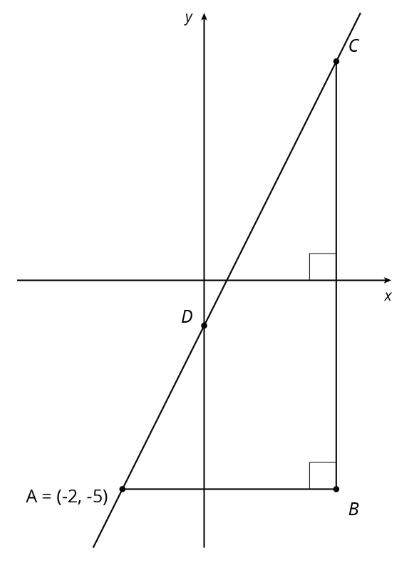What are the coordinates of B?

(x,y)

with no spaces.

Part B)

What are the coordinates of C?

(x,y)

with no spaces.

Part C)

What are the coordinates of D?

(x,y)

with no spaces.

Part A)

Match each graph of a linear relationship to a situation that most reasonably reflects its context.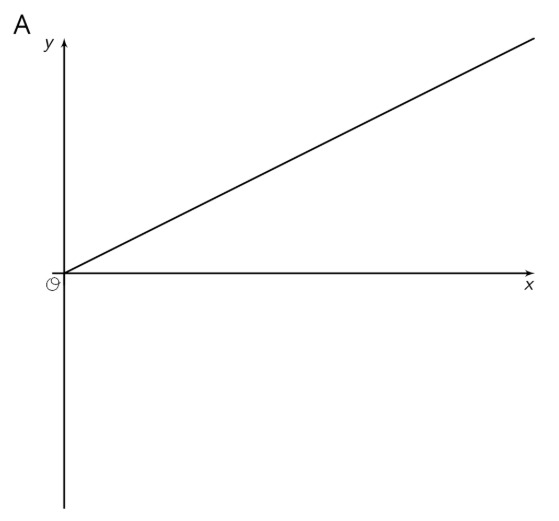Select one:
Part B)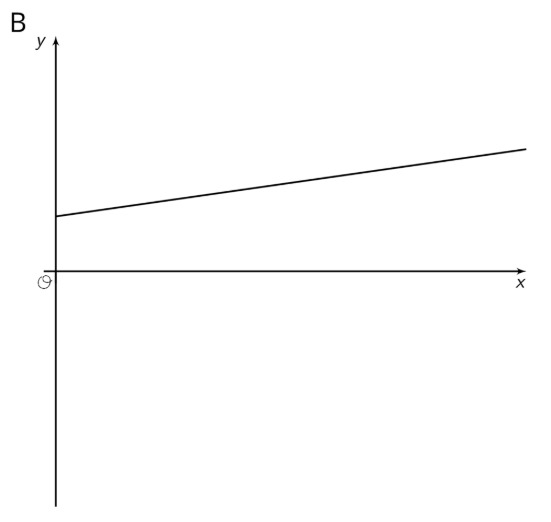Select one:
Part C)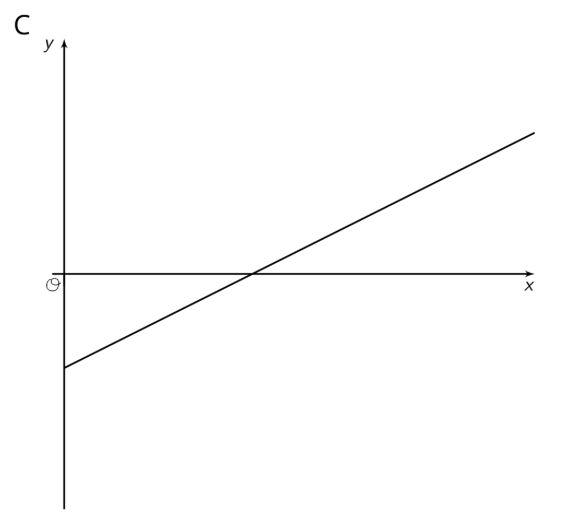Select one:
Part D)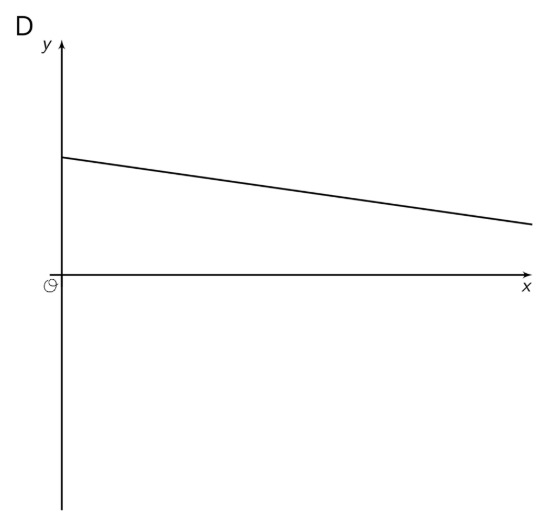Select one: